# Nickzom Calculator Calculates the Capacitive Reactance of a Simple AC Circuit

According to Electronics Tutorials,

Capacitors that are connected to a sinusoidal supply produce reactance from the effects of supply frequency and capacitor size.

The capacitive reactance of the capacitor decreases as the frequency across it increases therefore capacitive reactance is inversely proportional to frequency.

The opposition to current flow, the electrostatic charge on the plates (its AC capacitance value) remains constant as it becomes easier for the capacitor to fully absorb the change in charge on its plates during each half cycle.

In Physics, Nickzom Calculator solves a whole lot of calculations and thereby relieves the stress on scientists. One of those calculations is on Simple AC Circuit

Today, I am sharing with you how you can find answers to computations on Capacitive Reactance.

First and Foremost, Proceed to the Calculator Map, then scroll to the Physics section and click on Simple A.C. Circuit.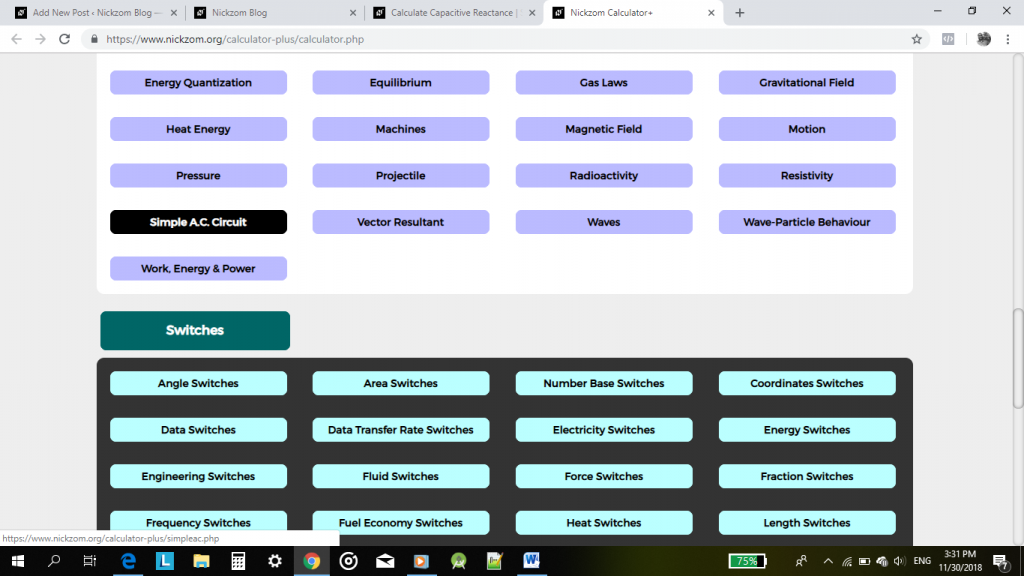Now, click on Capacitive Reactance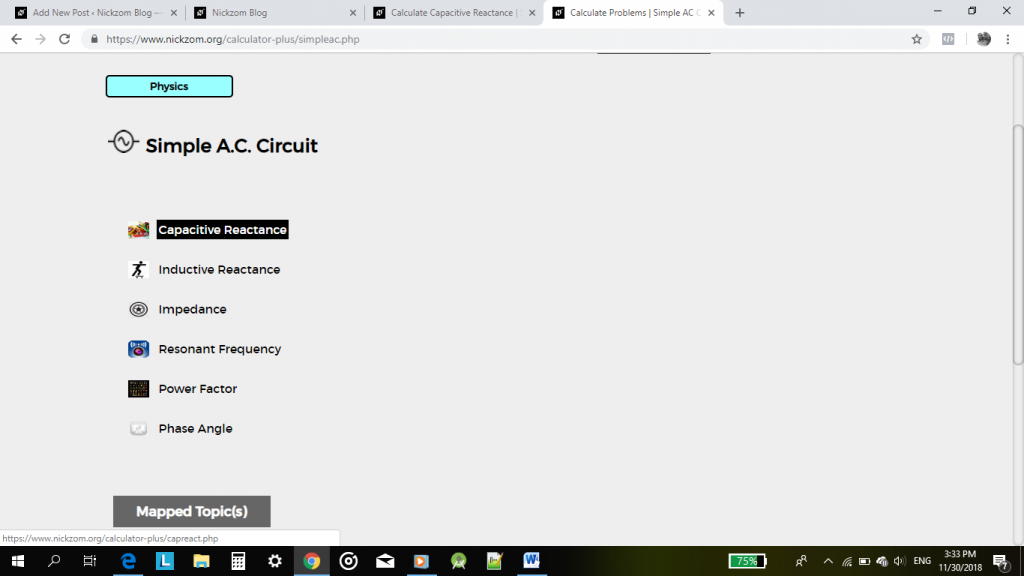An activity or page is displayed for you to enter the required parameters to compute the capacitive reactance which are:

• Frequency
• Capacitance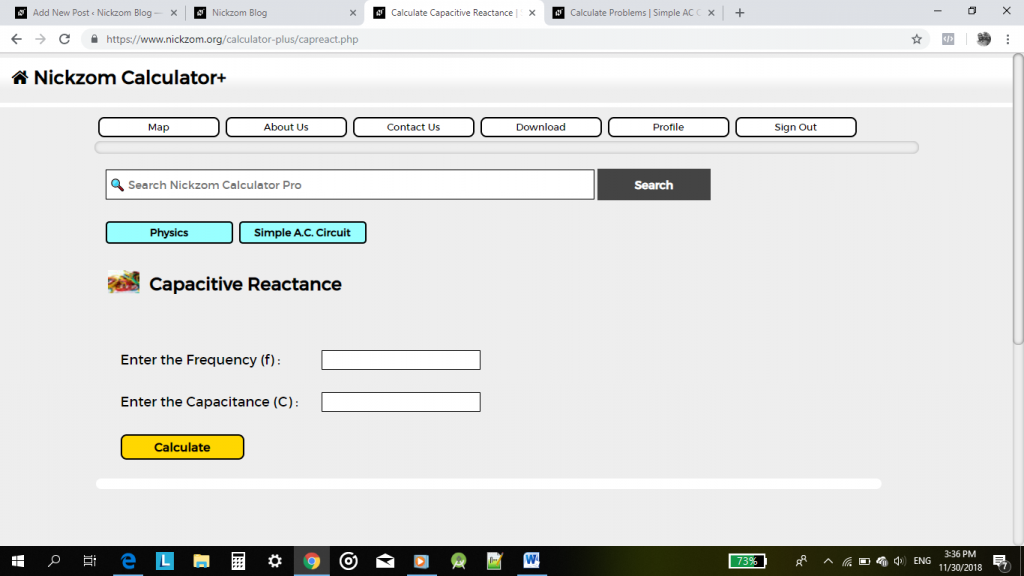Now, enter the appropriate values of the parameters accordingly.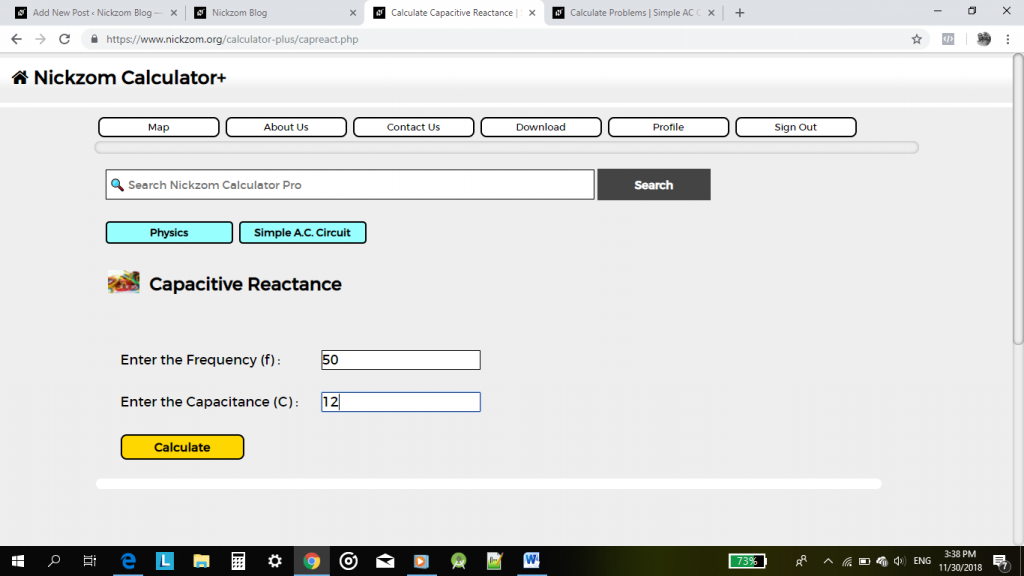Lastly, click on Calculate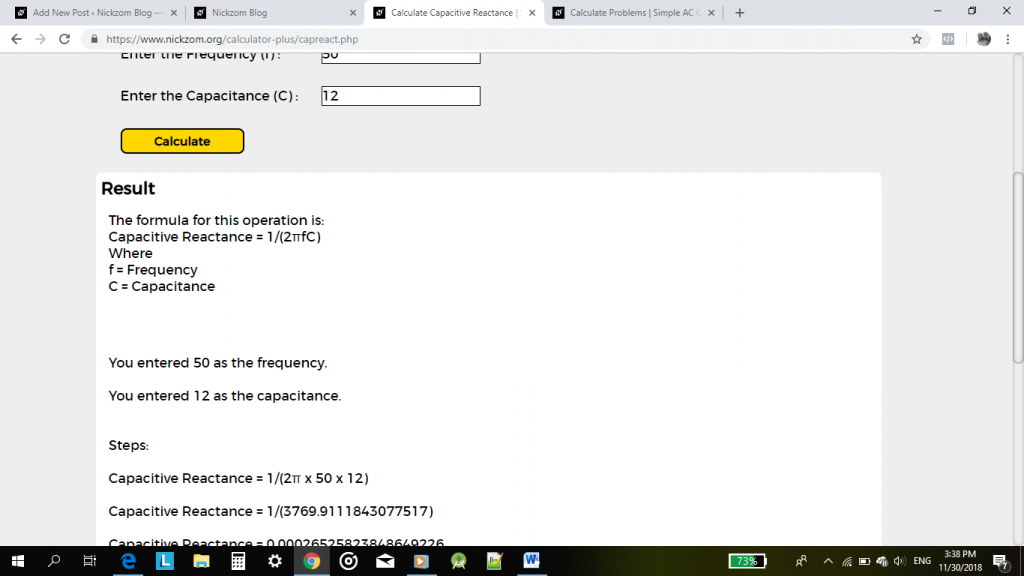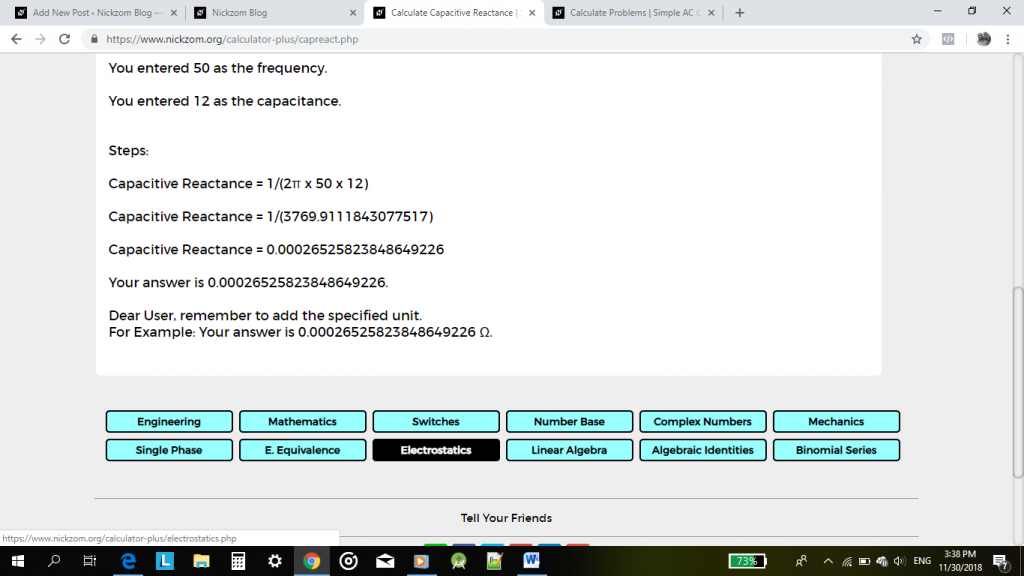THE END!

To access all the functionalities in Nickzom Calculator, you need to purchase our professional version – Nickzom Calculator+ (The Calculator Encyclopedia). You can access this software via any of these channels: